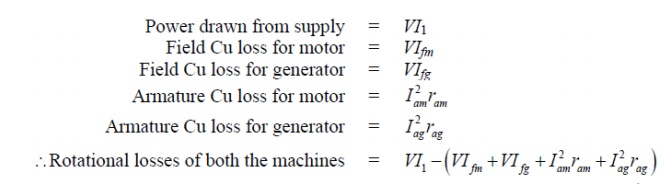Home | | Electrical Machines I | HopkinsonŌĆÖs test: Procedure, Calculation of efficiency, Advantages, Disadvantages

## Chapter: Electrical machines : DC Motor

This as an elegant method of testing d.c machines. Here it will be shown that while power drawn from the supply only corresponds to no load losses of the machines, the armature physically carries any amount of current (which can be controlled with ease).

HopkinsonŌĆÖs test

This as an elegant method of testing d.c machines. Here it will be shown that while power drawn from the supply only corresponds to no load losses of the machines, the armature physically carries any amount of current (which can be controlled with ease). Such a scenario can be created using two similar mechanically coupled shunt machines. Electrically these two machines are eventually connected in parallel and controlled in such a way that one machine acts as a generator and the other as motor. In other words two similar machines are required to carry out this testing which is not a bad proposition for manufacturer as large numbers of similar machines are manufactured.## Procedure

Connect the two similar (same rating) coupled machines as shown in figure 40.6. With switch S opened, the first machine is run as a shunt motor at rated speed. It may be noted that the second machine is operating as a separately excited generator because its field winding is excited and it is driven by the first machine. Now the question is what will be the reading of the voltmeter connected across the opened switch S? The reading may be (i) either close to twice supply voltage or (ii) small voltage. In fact the voltmeter practically reads the difference of the induced voltages in the armature of the machines. The upper armature terminal of the generator may have either + ve or negative polarity. If it happens to be +ve, then voltmeter reading will be small otherwise it will be almost double the supply voltages

Since the goal is to connect the two machines in parallel, we must first ensure voltmeter reading is small. In case we find voltmeter reading is high, we should switch off the supply, reverse the armature connection of the generator and start afresh. Now voltmeter is found to read small although time is still not ripe enough to close S for paralleling the machines. Any attempt to close the switch may result into large circulating current as the armature resistances are small. Now by adjusting the field current Ifg of the generator the voltmeter reading may be adjusted to zero (Eg Ōēł Eb) and S is now closed. Both the machines are now connected in parallel

After the machines are successfully connected in parallel, we go for loading the machines i.e., increasing the armature currents. Just after paralleling the ammeter reading A will be close to zero as Eg Ōēł Eb. Now if Ifg is increased (by decreasing Rfg),  then  Eg becomes  greater  than  Eb  and  both  Iag  and  Iam  increase,  Thus  by increasing field current of generator (alternatively decreasing field current of motor) one can make Eg > Eb so as to make the second machine act as generator and first machine as motor. In practice, it is also required to control the field current of the motor Ifm to maintain speed constant at rated value. The interesting point to be noted here is that Iag and Iam do not reflect in the supply side line. Thus current drawn from supply remains small (corresponding to losses of both the machines). The loading is sustained by the output power of the generator running the motor and vice versa. The machines can be loaded to full load current without the need of any loading arrangement

## Calculation of efficiency

Let field currents of the machines be are so adjusted that the second machine is acting as generator with armature current Iag and the first machine is acting as motor with armature  current Iam as shown in figure 40.7. Also let us assume the current drawn from the supply be I1. Total power drawn from supply is VI1 which goes to supply all the losses (namely Cu losses in armature & field and rotational losses) of both the machinesSince speed of both the machines are same, it is reasonable to assume the rotational losses of both the machines are equal; which is strictly not correct as the field current of the generator will be a bit more than the field current of the motor, Thus, Once Prot is estimated for each machine we can proceed to calculate the efficiency of the machines as follows,## Efficiency of the motor

As pointed out earlier, for efficiency calculation of motor, first calculate the input power and then subtract the losses to get the output mechanical power as shown below,## EFFICIENCY OF GENERATORThe  merits         of      this    test    areŌĆ”

1. This test requires very small power compared to full-load power of the motor-generator coupled system. That is why it is economical.

2. Temperature rise and commutation can be observed and maintained in the limit because this test is done under full load condition.

3. Change in iron loss due to flux distortion can be taken into account due to the advantage of its full load condition

The demerits of this test are

1. It is difficult to find two identical machines needed for Hopkinson's test. 2. Both machines cannot be loaded equally all the time.

3. It is not possible to get separate iron losses for the two machines though they are different because of their excitations.

4. It is difficult to operate the machines at rated speed because field currents vary widely.

Study Material, Lecturing Notes, Assignment, Reference, Wiki description explanation, brief detail
Electrical machines : DC Motor : HopkinsonŌĆÖs test: Procedure, Calculation of efficiency, Advantages, Disadvantages |